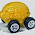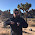Saturday, April 25, 2015

Economic potentials or: How to define an economy

I'm attempting to construct the thermodynamic potential for an economy by elaborate analogy -- demand/output is analogous to energy, price to pressure and supply to volume. What does this help with? For one thing, it leads toward a way to introduce a chemical potential (which after writing this post, I realize might not be necessary). However, it also allows for a way to organize thought around microeconomic and macroeconomic forces (see e.g. here or here).

Using the definitions here and here (and writing $N$ for nominal output, $X$ for goods with price $p$ -- which could be taken to be  the price level $P$ but we'll leave separate for now, $T$ for the 'economic temperature' and $S$ for the 'economic entropy' -- the latter two being defined at the links), we have for a monetary economy:

$$N = TS + \kappa P M + \alpha p X$$

$$N \approx c N/\kappa + \kappa P M + \alpha p X$$

where we use Stirling's approximation (with large $N$, but small changes) and the definitions

$$S \sim \log N! \approx N \log N - N \;\;\text{ and }\;\; 1/T \sim \log M$$

with $M$ being the money supply (empirically, base money minus reserves) and $\kappa = \kappa (N, M)$ being the information transfer index for the money market. Note that $\kappa \sim 1/T$ so that high $\kappa$ represents a low temperature economy and vice versa.

For multiple goods we have 

$$N = T S + \kappa P M + \sum_{i} \alpha_{i} p_{i} X_{i}$$

where the sum is over the individual market "generalized forces" (microeconomic forces). For example, we can look at a simple model of an aggregate goods market $A$ and a labor market $L$:

$$N = T S + \kappa P M + \alpha P A + \beta P L$$

... all prices for labor and goods are taken to be proportional to the price level. This allows us to organize microeconomic and macroeconomic forces

$$N = \underbrace{T S + \kappa P M}_{\text{macro forces}} + \underbrace{\alpha P A + \beta P L}_{\text{micro forces}}$$

In truth, the $P M$ component should probably be considered a microeconomic force (since it behaves like one for the most part) and only $TS$ -- the entropic forces -- should be considered macroeconomic forces. However, since $P M$ is a large component of the economy (and would likely be for a commodity money system as well, see footnote ) and policy-relevant, I'll keep it in. Understanding this distinction would point towards (using the separation from this earlier post about a financial and government sectors $F$ and $G$):

$$\text{(1) } N = T S + \kappa P M + \alpha P A + \beta P L + \gamma P G + \epsilon i F$$

where $i$ is a general market index (e.g. the S&P500 could be used). This approach can be compared with the older approaches that use the definition of nominal output:

$$N = C + I + G + NX$$

where we'd instead write (for example, assuming the prices are all proportional to the price level $P$):

$$\text{(2) }N = a_{1} P C + a_{2} P I + a_{3} P G + a_{4} P NX$$

The $a_{i}$ are all constants. Comparing equations (1) and (2) we can see that they mostly just represent different partitions of nominal output. Equation (2) lacks an explicit monetary component, but the biggest difference is that it lacks an 'entropic' component $T S$. I'd visualize $T S$ as the additional gains in welfare from exchange -- exchange makes both parties better off and increases the value of whatever it is that is exchanged.

Another topic that becomes clearer with the construction (1) is that of monetary vs Keynesian takes on macroeconomic stabilization. In (1), it becomes clear that a change in $G$ could be offset by a change in the $\kappa P M$ term or even the $T S$ term in general. In practice it depends on the details of the model (specifically the value of $\kappa$ -- if it is near 1 changes in $M$ have limited impact, and if it is near 1/2 you have an almost ideal quantity theory of money).

Additionally, the conditions that allow monetary offset of fiscal stimulus to occur also allow the monetary offset of the effects of a financial crisis. At least if (1) is a valid way to build an economy.

This last piece is interesting -- it implies that financial crises cause bigger problems in a liquidity trap economy ($\kappa \sim 1$). Assuming the model is correct, the reason the global financial crisis was so bad was because it struck when $\kappa \sim 1$ for a large portion of the world economy: the EU, US, and Japan. Other financial crises (e.g. 1987 in the US or even the dot-com boom) struck at a time when $\kappa < 1$ and were better able to be offset by monetary policy.

Footnotes:

 Actually, the $\kappa P M$ component is like one of the goods markets and in e.g. a commodity money economy, it would be one (and entropy should be defined in terms of that good). However it may be more useful to separate it as a macroeconomic force as is done later in the post.

1.I'm not sure I understand why TS should be consider gains in welfare...this increase in "value" you mention could just be a signal of say a house mortgage as being good currency for stocks and any welfare could be only through the agents' expectations of value from the house payments or selling the stock...

1.The T*S term comes from the large number of exchanges in an economy ... More technically it represents the generalized force against undoing moves towards equilibrium (and is maximized at equilibrium). I interpreted this as a lack of desire to undo utility gains from exchange, but as even mindless atoms in this system would do the same thing it's probably not useful to put any kind of behavioral interpretation on it :)

2.This comment has been removed by the author.

3.It's funny to see you going in cold turkey and attempting to do the derivation; anyway I started an Hmolpedia article on you:

http://www.eoht.info/page/Jason+Smith

http://www.eoht.info/page/Human+thermodynamics+variables+table

and to scrap Shannon.

1.Cheers.

That is an interesting collection of work.

2.In looking at your paper on information theory != thermodynamics, I'm not sure I understand why it is important to drive home the distinction between thermodynamics and information theory.

Information theory is thermodynamics without the conservation laws (symmetries like time and space translation). However, if we put on the blackboard the first day of thermodynamics class:

"information theory isn't a useful way to think about thermodynamics"

what do we gain? Let's say some student reads that on the blackboard and says:

"Oh! I see, I would have thought X using information theory as a frame, but now I see Y."

What are X and Y?